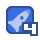# 输液控制报警系统设计（ULN2003-光耦）-电路方案

VIP专享 2021-04-20 09:32:34 247KB ZIP10收藏

#include #define uint unsigned int #define uchar unsigned char sbit beep = P2^0; //步进电机步进数组 uchar code CCW={0x80,0xc0,0x40,0x60,0x20,0x30,0x10,0x90}; //逆时钟旋转相序表 uchar code CWW={0x90,0x10,0x30,0x20,0x60,0x40,0xc0,0x80}; //正时钟旋转相序表 uchar FZ=0;//0不转 1正转 2反转 uchar SD=0;//0，1，2，3，4速度级别 uchar BB=0; //步 //电机接口 #define JK P1 uchar qian,bai,shi,ge; uint speed = 0,speed_tmp = 0; uint num0,num1; uchar time_out = 0; uchar code TAB[]={0xc0,0xcf,0xa4,0xb0,0x99,0x92,0x82,0xf8,0x80,0x90}; uchar code TAB1[]={0xbf,0x89,0xc7,0xa7}; //三个依次是 - H L 后面两个是温度符号 sbit wei1 = P2^1; //数码管位选 sbit wei2 = P2^2; sbit wei3 = P2^3; sbit wei4 = P2^4; bit yyp=1; uchar s1num=0; uint htemp=96,ltemp=48;//上下线初始化 void init(); void delayms(uint); void display(uchar speed_tmp); /****毫秒级延时函数*****/ sbit k1=P3^4;//功能按键 sbit k2=P3^5; // 增加键 sbit k3=P3^6;//减少建 void delay(uint x) { uint i,j; for(i=x;i>0;i--) for(j=110;j>0;j--); } void delay_T(uchar T) //误差 0us { uint a,b; for(b=T*2+10;b>0;b--) for(a=12;a>0;a--); } void di() { beep=0; delay(300); beep=1; } //步进电机处理函数 void BJDJ(uchar FZ,uchar SD) { if(FZ==1) { JK=CCW[BB]; BB++; delay_T(SD*6); if(BB==8) { BB=0; } } else if(FZ==2) { JK=CWW[BB]; BB++; delay_T(SD*6); if(BB==8) { BB=0; } } else { JK=0x00; } } /////////调最高调用的函数////// void hdisplay(uchar dat) { uchar fs,fg,fb; wei1=0; //符号“H-” P0=TAB1; delay(5); wei1=1; P0=0xff; fb=dat/100; fs=dat/10; fg=dat; wei2=0; P0=TAB[fb]; delay(5); wei2=1; P0=0xff; wei3=0; P0=TAB[fs]; delay(5); wei3=1; P0=0xff; wei4=0; P0=TAB[fg]; delay(5); wei4=1; P0=0xff; } /////////调最低调用的函数////// void ldisplay(uchar dat) { uchar fs,fg,fb; wei1=0; //符号“L=” P0=TAB1; delay(2); wei1=1; P0=0xff; fb=dat/100; fs=dat/10; fg=dat; wei2=0; P0=TAB[fb]; delay(2); wei2=1; P0=0xff; wei3=0; P0=TAB[fs]; delay(2); wei3=1; P0=0xff; wei4=0; P0=TAB[fg]; delay(2); wei4=1; P0=0xff; } /****按键扫描******/ void keyscan() { if(k1==0) { delay(10); if(k1==0) { yyp=0; s1num++; while(!k1);//等待按键释放 di(); switch(s1num) { case 1:break; c

...展开详情

• 647
资源
• 3
粉丝
•等级
•至尊王者输液控制报警系统设计（ULN2003-光耦）-电路方案 (VIP专享) VIP下载
1/0